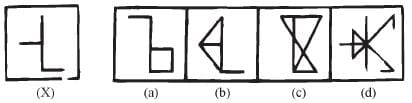Olympiad Test: Embedded Figure - 1

# Olympiad Test: Embedded Figure - 1

Test Description

## 20 Questions MCQ Test Science Olympiad Class 6 | Olympiad Test: Embedded Figure - 1

Olympiad Test: Embedded Figure - 1 for Class 6 2022 is part of Science Olympiad Class 6 preparation. The Olympiad Test: Embedded Figure - 1 questions and answers have been prepared according to the Class 6 exam syllabus.The Olympiad Test: Embedded Figure - 1 MCQs are made for Class 6 2022 Exam. Find important definitions, questions, notes, meanings, examples, exercises, MCQs and online tests for Olympiad Test: Embedded Figure - 1 below.
 1 Crore+ students have signed up on EduRev. Have you?
Olympiad Test: Embedded Figure - 1 - Question 1

### Directions: Analyze the set of figures and choose the correct option that contains figure X.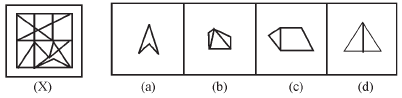Olympiad Test: Embedded Figure - 1 - Question 2

### Directions: Analyze the set of figures and choose the correct option that contains figure X.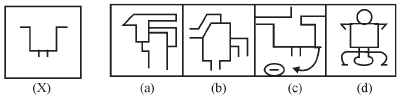Olympiad Test: Embedded Figure - 1 - Question 3

### Directions: Analyze the set of figures and choose the correct option that contains figure X.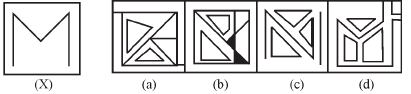Olympiad Test: Embedded Figure - 1 - Question 4

Directions: Analyze the set of figures and choose the correct option that contains figure X.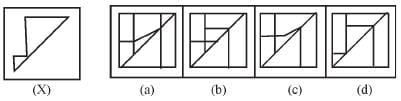Olympiad Test: Embedded Figure - 1 - Question 5

Directions: Analyze the set of figures and choose the correct option that contains figure X.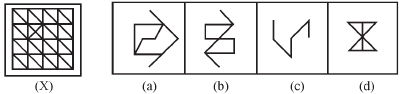Olympiad Test: Embedded Figure - 1 - Question 6

Directions: Analyze the set of figures and choose the correct option that contains figure X.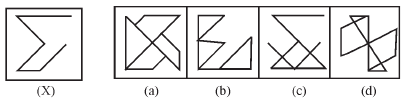Olympiad Test: Embedded Figure - 1 - Question 7

Directions: Analyze the set of figures and choose the correct option that contains figure X.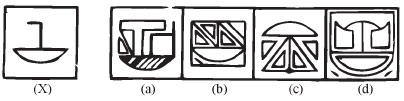Olympiad Test: Embedded Figure - 1 - Question 8

Directions: Analyze the set of figures and choose the correct option that contains figure X.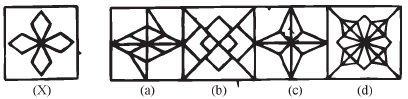Olympiad Test: Embedded Figure - 1 - Question 9

Directions: Analyze the set of figures and choose the correct option that contains figure X.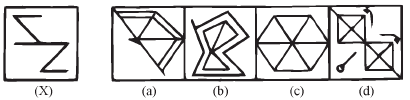Olympiad Test: Embedded Figure - 1 - Question 10

Directions: Analyze the set of figures and choose the correct option that contains figure X.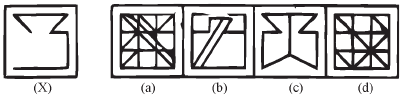Olympiad Test: Embedded Figure - 1 - Question 11

Directions: Analyze the set of figures and choose the correct option that contains figure X.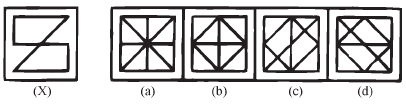Olympiad Test: Embedded Figure - 1 - Question 12

Directions: Analyze the set of figures and choose the correct option that contains figure X.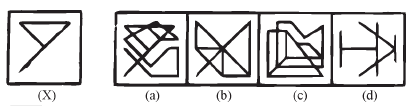Olympiad Test: Embedded Figure - 1 - Question 13

Directions: Analyze the set of figures and choose the correct option that contains figure X.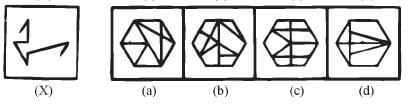Olympiad Test: Embedded Figure - 1 - Question 14

Directions: Analyze the set of figures and choose the correct option that contains figure X.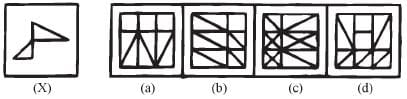Olympiad Test: Embedded Figure - 1 - Question 15

Directions: Analyze the set of figures and choose the correct option that contains figure X.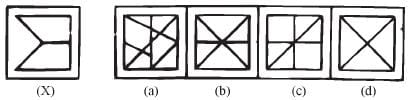Olympiad Test: Embedded Figure - 1 - Question 16

Directions: Analyze the set of figures and choose the correct option that contains figure X.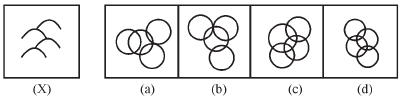Olympiad Test: Embedded Figure - 1 - Question 17

Directions: Analyze the set of figures and choose the correct option that contains figure X.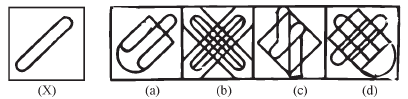Olympiad Test: Embedded Figure - 1 - Question 18

Directions: Analyze the set of figures and choose the correct option that contains figure X.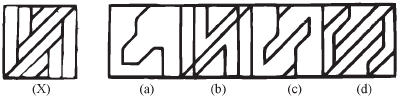Olympiad Test: Embedded Figure - 1 - Question 19

Directions: Analyze the set of figures and choose the correct option that contains figure X.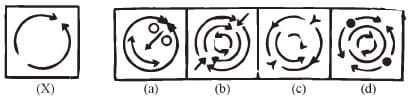Olympiad Test: Embedded Figure - 1 - Question 20

Directions: Analyze the set of figures and choose the correct option that contains figure X.